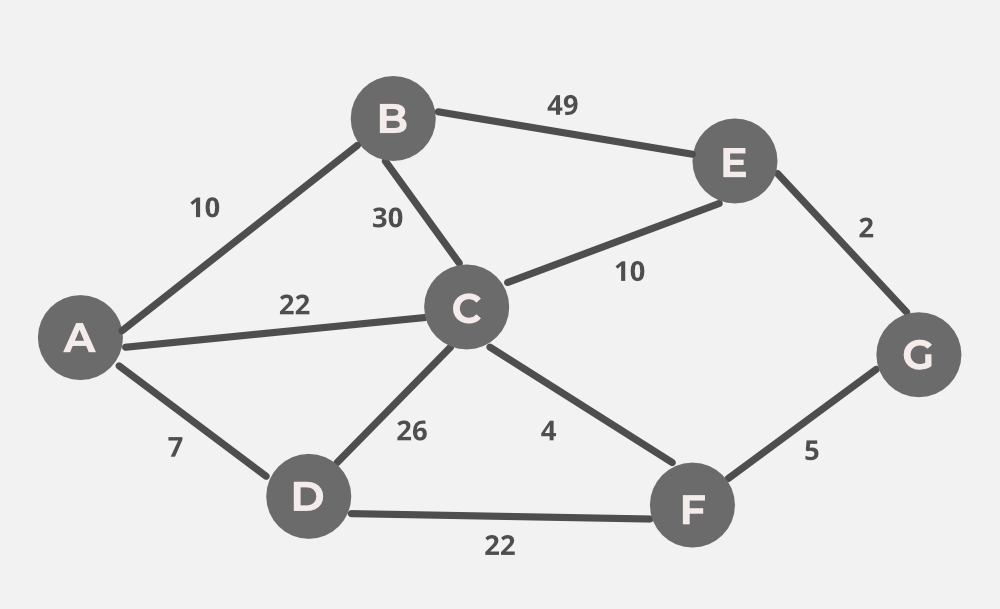# GATE | GATE-IT-2004 | Question 56

Consider the undirected graph below:Using Prim’s algorithm to construct a minimum spanning tree starting with node A, which one of the following sequences of edges represents a possible order in which the edges would be added to construct the minimum spanning tree?
(A) (E, G), (C, F), (F, G), (A, D), (A, B), (A, C)
(B) (A, D), (A, B), (A, C), (C, F), (G, E), (F, G)
(C) (A, B), (A, D), (D, F), (F, G), (G, E), (F, C)
(D) (A, D), (A, B), (D, F), (F, C), (F, G), (G, E)

Explanation: A. False The idea behind Prim’s algorithm is to construct a spanning tree – means all vertices must be connected but here vertices are disconnected

B. False The idea behind Prim’s algorithm is to construct a spanning tree – means all vertices must be connected but here vertices are disconnected

C. False. Prim’s is a greedy algorithm and At every step, it considers all the edges that connect the two sets, and picks the minimum weight edge from these edges. In this option weight of AB<AD so must be picked up first.

D.TRUE.